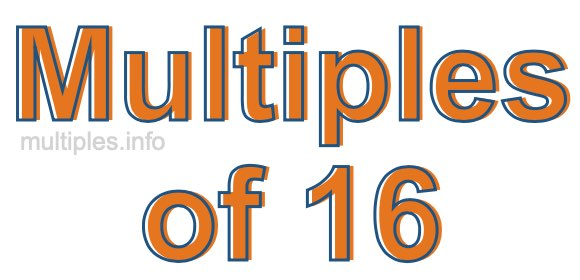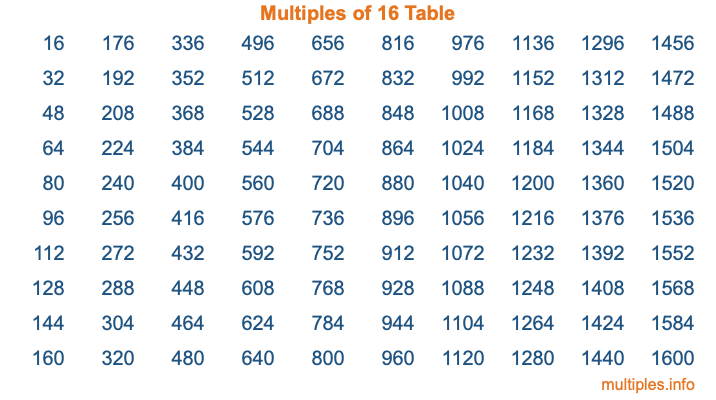Multiples of 16Welcome to the Multiples of 16 page. Here we will first teach you everything you will ever need to know about the multiples of 16, and then give you a study guide summary of everything we taught you to make sure you remember it all. Use this page to look up facts and learn information about the multiples of 16. This page will make you a multiples of sixteen expert!

Definition of Multiples of 16
Multiples of 16 are all the numbers that when divided by 16 equal an integer. Each of the multiples of 16 are called a multiple. A multiple of 16 is created by multiplying 16 by an integer.

Therefore, to create a list of multiples of 16, you start with 1 multiplied by 16, then 2 multiplied by 16, then 3 multiplied by 16, and so on for as long as you want. Thus, the list of the first five multiples of 16 is 16, 32, 48, 64, and 80. To see a larger list of multiples of 16, see the printable image of Multiples of 16 further down on this page. We also have a category where you can choose any nth multiple of 16.

Multiples of 16 Checker
The Multiples of 16 Checker below checks to see if any number of your choice is a multiple of 16. In other words, it checks to see if there is any number (integer) that when multiplied by 16 will equal your number. To do that, we divide your number by 16. If the the quotient is an integer, then your number is a multiple of 16.

Is  a multiple of 16?

Least Common Multiple of 16 and ...
A Least Common Multiple (LCM) is the lowest multiple that two or more numbers have in common. This is also called the smallest common multiple or lowest common multiple and is useful to know when you are adding our subtracting fractions. Enter one or more numbers below (16 is already entered) to find the LCM.

Check out our LCM Calculator if you need more details about the Least Common Multiple or if you need the LCM for different numbers for adding and subtraction fractions.

nth Multiple of 16
As we stated above, 16 is the first multiple of 16, 32 is the second multiple of 16, 48 is the third multiple of 16, and so on. Enter a number below to find the nth multiple of 16.

th multiple of 16

Multiples of 16 vs Factors of 16
16 is a multiple of 16 and a factor of 16, but that is where the similarities end. All postive multiples of 16 are 16 or greater than 16. All positive factors of 16 are 16 or less than 16.

Below is the beginning list of multiples of 16 and the factors of 16 so you can compare:

Multiples of 16: 16, 32, 48, 64, 80, etc.

Factors of 16: 1, 2, 4, 8, 16

As you can see, the multiples of 16 are all the numbers that you can divide by 16 to get a whole number. The factors of 16, on the other hand, are all the whole numbers that you can multiply by another whole number to get 16.

It's also interesting to note that if a number (x) is a factor of 16, then 16 will also be a multiple of that number (x).

Multiples of 16 vs Divisors of 16
The divisors of 16 are all the integers that 16 can be divided by evenly. Below is a list of the divisors of 16.

Divisors of 16: 1, 2, 4, 8, 16

The interesting thing to note here is that if you take any multiple of 16 and divide it by a divisor of 16, you will see that the quotient is an integer.

Multiples of 16 Table
Below is an image of the first 100 multiples of 16 in a table. The table is in chronological order, column by column. The first column has the first ten multiples of 16, the second column has the next ten multiples of 16, and so on.The Multiples of 16 Table is also referred to as the 16 Times Table or Times Table of 16. You are welcome to print out our table for your studies.

Negative Multiples of 16
Although not often discussed or needed in math, it is worth mentioning that you can make a list of negative multiples of 16 by multiplying 16 by -1, then by -2, then by -3, and so on, to get the following list of negative multiples of 16:

-16, -32, -48, -64, -80, etc.

Multiples of 16 Summary
Below is a summary of important Multiples of 16 facts that we have discussed on this page. To retain the knowledge on this page, we recommend that you read through the summary and explain to yourself or a study partner why they hold true.

There are an infinite number of multiples of 16.

A multiple of 16 divided by 16 will equal a whole number.

16 divided by a factor of 16 equals a divisor of 16.

The nth multiple of 16 is n times 16.

The largest factor of 16 is equal to the first positive multiple of 16.

16 is a multiple of every factor of 16.

16 is a multiple of 16.

A multiple of 16 divided by a divisor of 16 equals an integer.

16 divided by a divisor of 16 equals a factor of 16.

Any integer times 16 will equal a multiple of 16.

Multiples of a Number
Here you can get the multiples of another number, all with the same attention to detail as we did for multiples of 16 on this page.

Multiples of
Multiples of 17
Did you find our page about multiples of sixteen educational? Do you want more knowledge? Check out the multiples of the next number on our list!